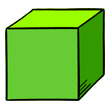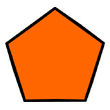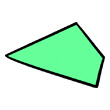Name:    2nd Grade Geometry  - Reason with shapes and their attrributes, Standard 1, Pre Test 1 Recognize and draw shapes having specified attributes, such as a given number of angles or a given number of equal faces.  Identify triangles, quadrilaterals, pentagons, hexagons, and cubes.

Multiple Choice
Identify the choice that best completes the statement or answers the question.

1.

Suzie had a piece of paper cut so that it had only three sides to it., Which type of shape did she have?
 a. hexagon c. triangle b. quadrilateral d. pentagon

2.

If Suzie has a a piece of paper with 4 equal sides and four right angles, which type of shape does she have?
 a. hexagon c. triangle b. quadrilateral d. pentagon

3.

Suzie cut out shapes from some colored paper.  One of the shapes had 5 sides to it.  What tyupe of shape did she have?
 a. hexagon c. triangle b. quadrilateral d. pentagon

4.

Which type of shape below has 6 sides?
 a. cube c. rectangles b. squares d. hexagon

5.Which shape is this?
 a. cube c. square b. pentagon d. hexagon

6.Which shape is this?
 a. cube c. square b. pentagon d. hexagon

7.Which shape is this?
 a. cube c. triangle b. pentagon d. hexagon

8.

Jenny took a toy out of the toybox.  She noticed that it had 6 angles to it.  What type of shape was the toy?
 a. hexagon c. triangle b. pentagon d. square

9.What type of shape is this?
 a. pyramid c. triangle b. quadrilateral d. rectangle

10.

What type of shape is the top of a shoe box?
 a. pentagon c. hexagon b. quadrilateral d. cube